# 深入學習 lsd-slam - 3

## 再訪 Direct Method

1. 是要定位相機 pose，並同時定位
2. 為達目的，這邊需要用影像資訊來估計 pose
3. 為了估計 pose，需要計算 photometric error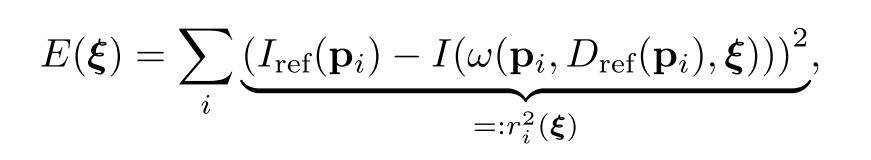## $\xi$、$\omega$ 是什麼?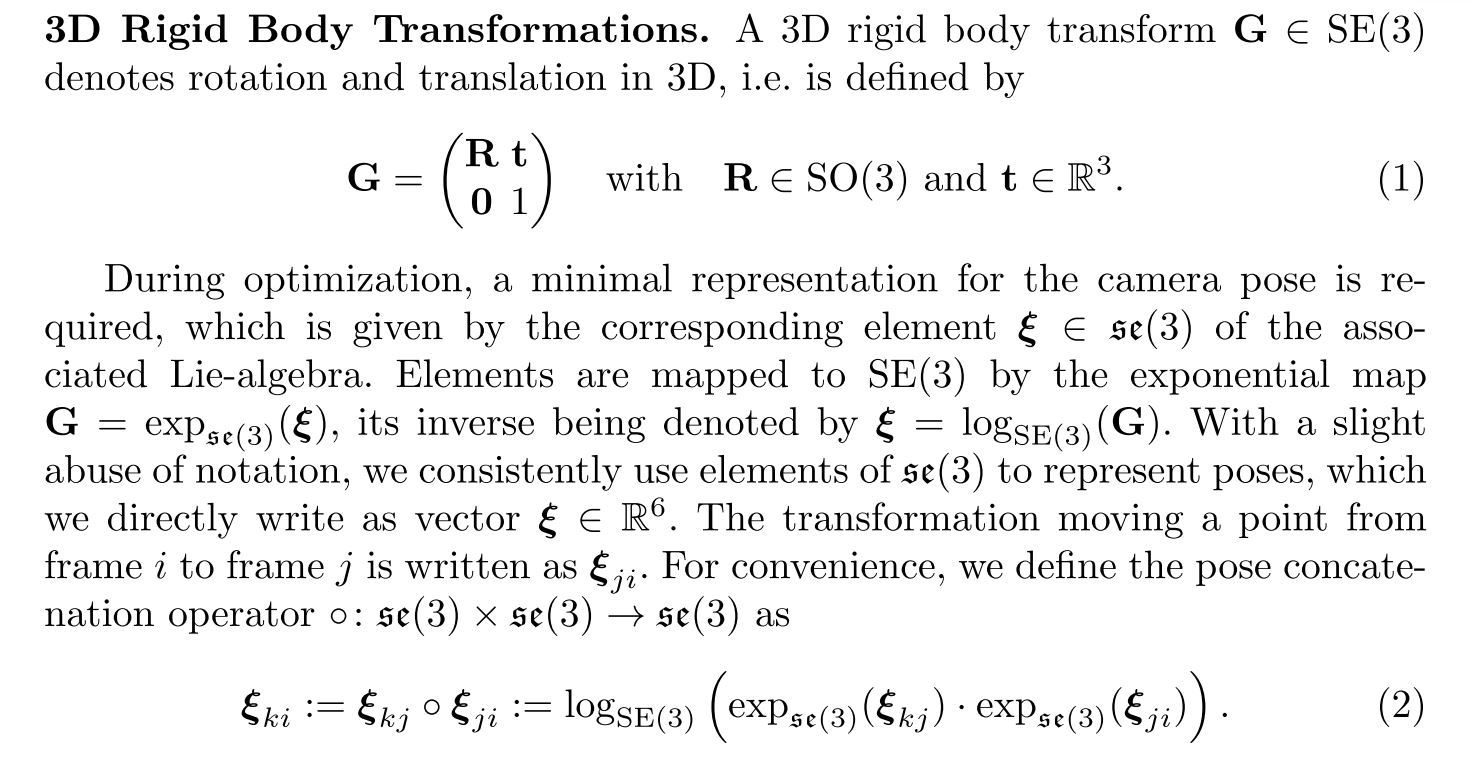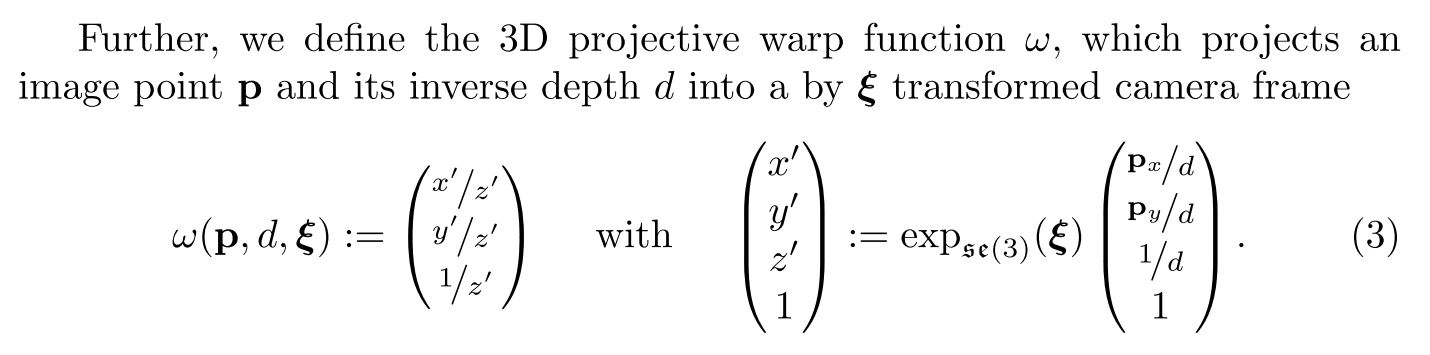## 再度深入 $\xi$

### 3D Rigid body transform

$G$ 就是一個可以對三維世界中的任意一點 $x$，做一個操作，使得 $x$ 點被轉換到 $x^\prime$：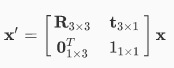### 李群與李代數

• 群：同一種東西的集合再加上同一種運算所構成的結構。記成 $(A,\cdot)$，要注意的是， $\cdot$ 運算需要滿足幾個條件，其中一個是封閉性：

$$\forall a_1, a_2, \quad a_1 \cdot a_2 \in A$$

• $SO(n)$：特殊正交群，也就是 $n$ 維空間上的旋轉矩陣構成的群。所以你可以想像，你手上有一群 $n \times n$ 的矩陣，每個都符合下式中旋轉矩陣的特性，那這一群矩陣，跟這群矩陣可以做的運算就構成了 $SO(n)$。

$\begin{equation} SO(n) = { \mathbf{R} \in \mathbb{R}^{n \times n} | \mathbf{R R}^T = \mathbf{I}, det(\mathbf{R})=1 } \end{equation}$

• $SE(n)$：概念上在 $SO(n)$ 的旋轉矩陣上加上一個平移向量。例如 $SE(3)$ 的定義如下。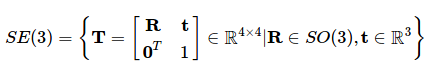• $\mathfrak{so}(3)$ 跟 $\mathfrak{se}(3)$：李代數，他的定義是在單位矩陣處的正切空間（tangent space），這很難簡單敘述，建議有興趣的讀者可以去看看 這篇文章，之後有需要我再仔細描述。

## 延伸閱讀

@pojenlai 演算法工程師，對機器人跟電腦視覺有少許研究，最近在鍛鍊自己的執行力